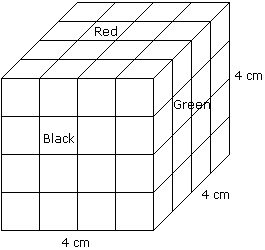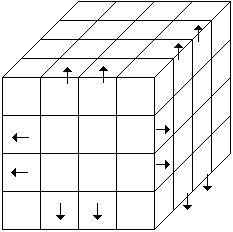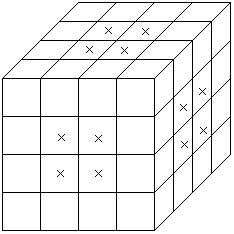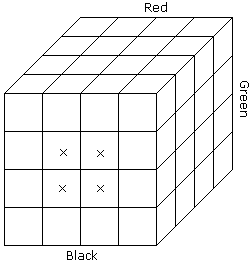# Verbal Reasoning - Cube and Cuboid

### Exercise :: Cube and Cuboid - Introduction

Intrducion:

• In a cube or a cuboid there are six faces in each.
• In a cube length, breadth and height are same while in cuboid these are different.
• In a cube the number of unit cubes = (side)3.
• In cuboid the number of unit cube = (l x b x h).

Example:

A cube of each side 4 cm, has been painted black, red and green on pars of opposite faces. It is then cut into small cubes of each side 1 cm.The following questions and answers are based on the information give above:

1. How many small cubes will be there ?

Total no. of cubes = (sides)3 = (4)3 = 64

2. How many small cubes will have three faces painted ?

From the figure it is clear that the small cube having three faces coloured are situated at the corners of the big cube because at these corners only three faces of the big cube meet.

Therefore the required number of such cubes is always 8, because there are 8 corners.

3. How many small cubes will have only two faces painted ?

From the figure it is clear that to each edge of the big cube 4 small cubes are connected and two out of them are situated at the corners of the big cube which have all three faces painted.

Thus, to edge two small cubes are left which have two faces painted. As the total no. of edges in a cube are 12.

Hence the no. of small cubes with two faces coloured = 12 x 2 = 24

(or)

No. of small cubes with two faces coloured = (x - 2) x No. of edges

where x = (side of big cube / side of small cube)4. How many small cubes will have only one face painted ?

The cubes which are painted on one face only are the cubes at the centre of each face of the big cube.

Since there are 6 faces in the big cube and each of the face of big cube there will be four small cubes.

Hence, in all there will be 6 x 4 = 24 such small cubes (or) (x - 2)2 x 6.5. How many small cubes will have no faces painted ?

No. of small cubes will have no faces painted = No. of such small cubes

= (x - 2)3 [ Here x = (4/1) = 4 ]

= (4 - 2)3

= 8.

6. How many small cubes will have only two faces painted in black and green and all other faces unpainted ?

There are 4 small cubes in layer II and 4 small cubes in layer III which have two faces painted green and black.

Required no. of such small cubes = 4 + 4 = 8.

7. How many small cubes will have only two faces painted green and red ?

No. of small cubes having two faces painted green and red = 4 + 4 = 8.

8. How many small cubes will have only two faces painted black and red ?

No. of small cubes having two faces painted black and red = 4 + 4 = 8.

9. How many small cubes will have only black painted ?

No. of small cubes having only black paint. There will be 8 small cubes which have only black paint. Four cubes will be form one side and 4 from the opposite side.

10. How many small cubes will be only red painted ?

No. of small cubes having only red paint = 4 + 4 = 8.11. How many small cubes will be only green painted ?

No. of small cubes having only green paint = 4 + 4 = 8.

12. How many small cubes will have at least one face painted ?

No. of small cubes having at least one face painted = No. of small cubes having 1 face painted + 2 faces painted + 3 faces painted

= 24 + 24 + 8

= 56.

13. How many small cubes will have at least two faces painted ?

No. of small cubes having at least two faces painted = No. of small cubes having two faces painted + 3 faces painted

= 24 + 8

= 32.